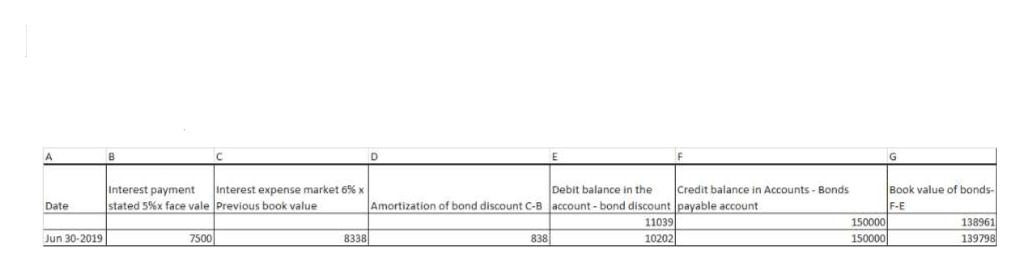# On January 1, 2019, Canglon, Inc., issues 10%, 5-year bonds with a face value of \$150,000 when the effective rate is 12%. Interest is to be paid semiannually on June 30 and December 31. Assume Canglon uses the effective interest method to amortize the discount.Required: Prepare the journal entry to record the first interest payment on June 30, 2019.

Question
39 views
On January 1, 2019, Canglon, Inc., issues 10%, 5-year bonds with a face value of \$150,000 when the effective rate is 12%. Interest is to be paid semiannually on June 30 and December 31. Assume Canglon uses the effective interest method to amortize the discount.
Required:
 Prepare the journal entry to record the first interest payment on June 30, 2019.
check_circle

star
star
star
star
star
1 Rating
Step 1

First of all, we have the below information-

Face value of bonds= \$150,000

Time of the bonds= 5 years

Interest paid semi annually = therefore, 5x 2 times a year= 10 payments

Interest rate of bonds= 10%, therefore 5%  semi annually.

Effective rate or market rate of bonds= 12% , therefore 6%  semi annually.

Step 2

We shall calculate the Actual interest to be paid = 150,000 x 5% = 7500 (10 payments after every 6mnths)

Now, we shall calculate the present value of bonds and interest using the market/effective rate of 6%

We can use the present value table for \$1 for calculation of bonds face value & Present value of ordinary annuity table for calculation of interest on bonds with effective rate-

Present value of Bonds of face value \$150000 = 150000 x 0.5584= \$83,761

Present value of interest of \$ 45000 = 7500 x 7.3601= \$55,200

Total present value of bonds= \$ 83760 + \$ 55200= \$ 138961

(please note present values have been picked up from the present value and annuity tables where rate = 6% and n= 10 years

Step 3

Using the figures arrived in step 2,  we shall now prepare the attached table. Please note-

1. Book value in Column G & Bond discount in column E  is as per calculated in ste...help_outlineImage TranscriptioncloseInterest payment stated 5%x face vale Previous book value Credit balance in Accounts - Bonds Debit balance in the Book value of bonds- Interest expense market 6% x F-E 150000 150000 Amortization of bond discount C-B account - bond discount payable account Date 11039 10202 138961 Jun 30-2019 8338 838 7500 139798 fullscreen

### Want to see the full answer?

See Solution

#### Want to see this answer and more?

Solutions are written by subject experts who are available 24/7. Questions are typically answered within 1 hour.*

See Solution
*Response times may vary by subject and question.
Tagged in